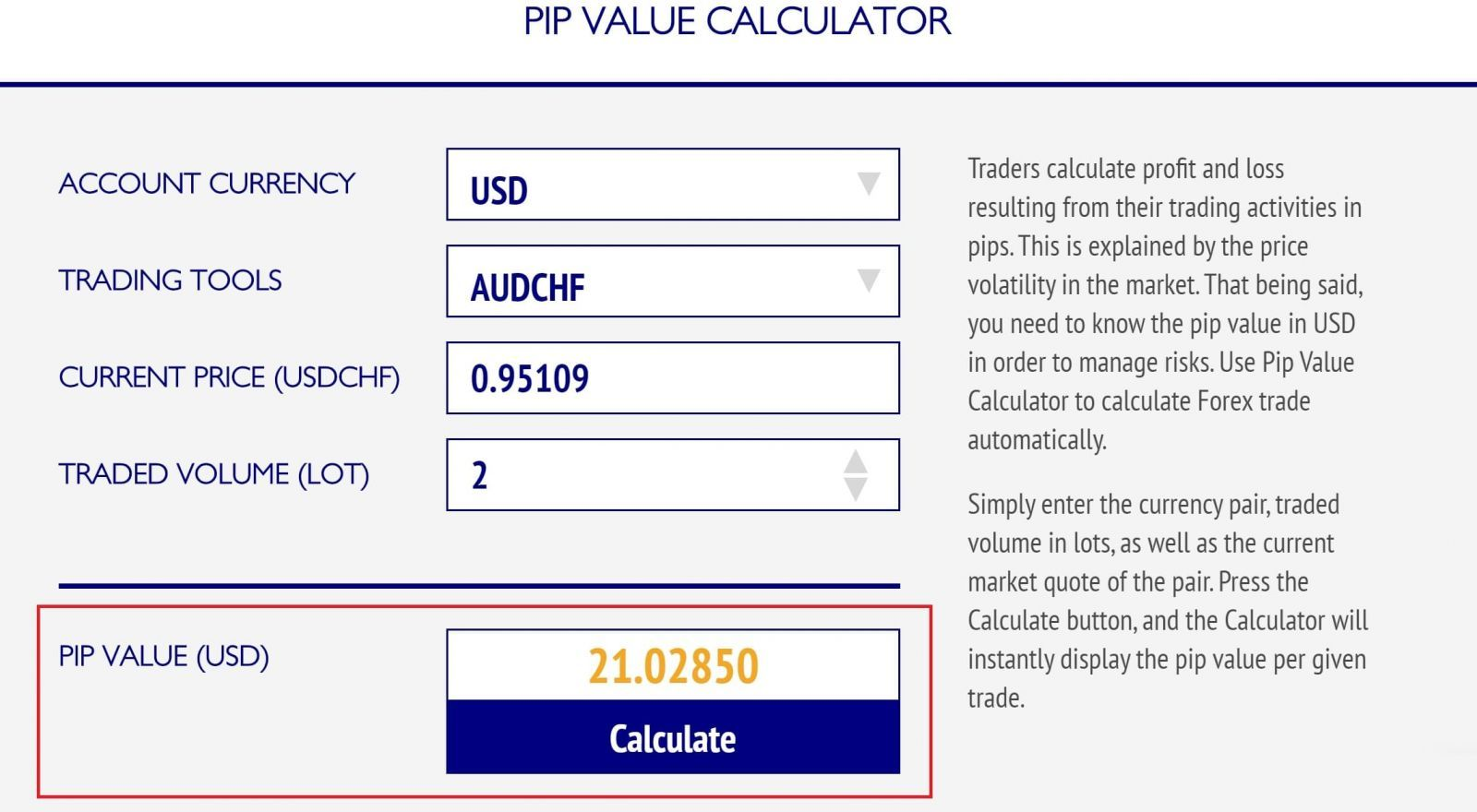# A guide to using trader’s calculatorThe topic of today’s article is Trader’s Calculator. Smart money management is one of the key pillars of successful trading, and Trader’s Calculator is just the tool every trader needs.

It is designed to calculate both risks and money accurately. On top of that, it allows moving away from manual calculations which - you have to admit - can be pretty exhausting sometimes. With Trader’s Calculator, risk management becomes quick and effortless. Read the article to learn how to use it correctly.

## Trader's Calculator: all calculations in one place

Before opening a position, the trader needs to calculate the volume for the trade entry, the pip value for the chosen trading instrument, the risk in the deposit currency, and other essential parameters. The online trading calculator tackles all these tasks. All you need to do is enter relevant data, and the system will generate the figures for you.

### How much does one pip cost

Essentially, a pip means is the smallest price move in the financial market. While we can determine the number of pips until the estimated take profit or stop loss on the chart using the cross line, the pip value in the deposit currency may vary for different pairs. It also changes depending on the volume you are planning to open a position with.

Using the Trader’s Calculator, you can calculate the pip value automatically. To do so, enter three parameters, namely the deposit currency, the trading instrument in question, and the desired position volume in the “Pip Value Calculator” window.Every trader knows - you cannot avoid the risks in the financial markets entirely. The good news is, the risks can still be managed. Given that each trading strategy can generate both profitable and losing trades, it is essential that the risk is smaller than the expected profit. This is why, rule number one in money management is to stick to the ratio of stop loss to take profit which is at least 1:2, and preferably 1:3 and higher.

Rule number two is this: the risk per trade in money terms should not exceed the percentage you’ve indicated. In the case of aggressive trading strategies, this parameter can reach up to 5%, and should not exceed 1% when it comes to conservative ones. Quickly converting this figure into the deposit currency after you have measured your stop loss in pips poses a certain challenge. But fear not because Trader’s Calculator comes to the rescue again.

Last but not least, the key parameter you need to know about when opening the trade is the volume (lot size) which you can open a position with. It is calculated based on the size of the stop loss, the deposit size and the allowable risk percentage per trade. We can calculate all of the above using the online Risk Calculator.

Here you can find such sections as the pip value (which we calculated previously), current balance, stop loss size in pips (which we learn from the chart), the traded volume in lots and the risk per trade in percentage terms.

Enter the available data in the Trader’s Calculator and mark the parameter you are interested in with a tick. Then simply press the “Calculate” button to learn the answer.Let’s assume that we are planning to trade EUR/USD pair with a stop loss of 25 pips. The allowable risk percentage here is 1%, with the pip value being \$10. Our deposit is \$10,000. We need to find out the allowable lot size per trade. To accomplish this, enter the above figures in the relevant sections of Trader's Calculator. The value we get is 0.40 lots.

In different types of trading accounts, the sizes of the minimum pip, trading lot and leverage are not the same. This means that the size of margin funds, the amount of potential profit in the deposit currency or the risk per trade change as well. These parameters can be quickly calculated in the “Trading Customization” section of the Trader’s Calculator.

E.g. you can calculate the margin size in the following manner. First of all, indicate the account type, account currency and leverage. Next, enter the trade parameters: currency pair, trade volume in lots, position direction (buy or sell), opening and closing prices. Press the “Calculate” button and you’ll know the size of the margin and profit. In this section, you can indicate not just one but several trades, and get the total result.In a matter of few minutes and even seconds, the online Trader’s Calculator will produce relevant figures. No need to fiddle with regular calculator and stress yourself anymore, for now you have an excellent tool at your fingertips to help calculate the parameters of a profitable trade.

## What to know:

Protect yourself against the trading risks

using Risk Manager brought to you by Gerchik & Co!